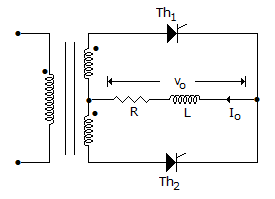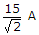# Electronics and Communication Engineering - Power Electronics

### Exercise :: Power Electronics - Section 3

41.

In a 3 phase semiconverter, the dc output voltage for a certain firing angle is 50% of the maximum possible value. The firing angle is

 A. 30° B. 60° C. 90° D. 120°

Explanation:

Vdc ∝ (1 + cos a). Maximum possible output voltage occurs when a = 0.

42.

A high frequency series inverter consists of

 A. a number of series inverters in series B. a number of series inverters in parallel C. a number of parallel inverters in parallel D. a number of parallel inverters in series

Explanation:

A high frequency series inverter has many series inverters in parallel. Only one inverter operates at one time and they operate in fixed sequence.

43.

In an SCR, the anode current consists of

 A. only holes B. only electrons C. both electrons and holes D. either electrons or holes

Explanation:

Both electrons and holes contribute to current.

44.

In the below figure, the average value of load current is 15 A. The load is highly inductive. The peak value of thyristor current isA. 15 A B. 152 A C.D. 30 A

Explanation:

Because of highly inductive load, output current is nearly constant.

45.

A 3 phase semiconverter when supplying a certain load has a rectification efficiency of 0.6 and transformer utilisation factor of 0.35. When a 3 phase full converter is supplying the same load

 A. rectification efficiency will be more than 0.6 and TUF will be more than 0.35 B. rectification efficiency will be more than 0.6 but TUF will be less than 0.35 C. rectification efficiency will be less than 0.6 and TUF will be less than 0.35 D. rectification efficiency will be less than 0.6 but TUF will be more than 0.35